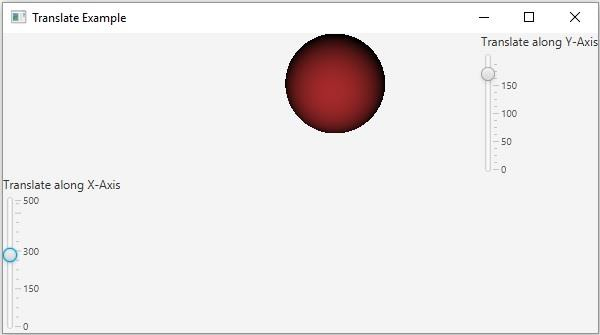# How to move (translate) a JavaFX node from one position to another?

JavaFXObject Oriented ProgrammingProgramming

If you move an object on the XY plane from one position to another it is known as translation. You can translate an object along either X-Axis to Y-Axis.

In JavaFX using the object of the javafx.scene.transform.Translate class you can translate a node from one position to another. This class contains three properties (double) representing the distance of the desired position from the original position along X, Y, Z plane respectively.

Every node in JavaFX contains an observable list to hold all the transforms to be applied on a node. You can get this list using the getTransforms() method.

To move a Node from one position to another −

• Instantiate the Translate class.

• Set the distance by which you want to move a node int the XYZ plane using the setX(), setY() and setZ() methods respectively.

• Get the list of transforms from the node (which you want to move) using the getTransforms() method.

• Add the above-created scale object to it.

• Add the node to the scene.

## Example

Following the JavaFX example demonstrates the translation transform. It contains a 2D geometric shape and two sliders, representing the x and y translate values. If you move the sliders the object moves along the chosen axis.

import javafx.application.Application;
import javafx.beans.value.ChangeListener;
import javafx.beans.value.ObservableValue;
import javafx.geometry.Orientation;
import javafx.scene.Scene;
import javafx.scene.control.Label;
import javafx.scene.control.Slider;
import javafx.scene.layout.BorderPane;
import javafx.scene.layout.VBox;
import javafx.scene.paint.Color;
import javafx.scene.paint.PhongMaterial;
import javafx.scene.shape.CullFace;
import javafx.scene.shape.DrawMode;
import javafx.scene.shape.Sphere;
import javafx.scene.transform.Translate;
import javafx.stage.Stage;
public class TranslateExample extends Application {
public void start(Stage stage) {
//Drawing a Sphere
Sphere sphere = new Sphere();
sphere.setCullFace(CullFace.BACK);
sphere.setDrawMode(DrawMode.FILL);
PhongMaterial material = new PhongMaterial();
material.setDiffuseColor(Color.BROWN);
sphere.setMaterial(material);
//Setting the slider for the horizontal translation
Slider slider1 = new Slider(0, 500, 0);
slider1.setOrientation(Orientation.VERTICAL);
slider1.setShowTickLabels(true);
slider1.setShowTickMarks(true);
slider1.setMajorTickUnit(150);
slider1.setBlockIncrement(150);
//Creating the translation transformation
Translate translate = new Translate();
//Linking the transformation to the slider
public void changed(ObservableValue <?extends Number>observable, Number oldValue, Number newValue){
translate.setX((double) newValue);
translate.setY(0);
translate.setZ(0);
}
});
//Setting the slider for the vertical translation
Slider slider2 = new Slider(0, 200, 0);
slider2.setOrientation(Orientation.VERTICAL);
slider2.setShowTickLabels(true);
slider2.setShowTickMarks(true);
slider2.setMajorTickUnit(50);
slider2.setBlockIncrement(50);
//Creating the translation transformation
public void changed(ObservableValue <?extends Number>observable, Number oldValue, Number newValue){
translate.setX(0);
translate.setY((double) newValue);
}
});
//Adding the transformation to the sphere
//Creating the pane
BorderPane pane = new BorderPane();
pane.setBottom(new VBox(new Label("Translate along X-Axis"), slider1));
pane.setRight(new VBox(new Label("Translate along Y-Axis"), slider2));
pane.setLeft(sphere);
//Preparing the scene
Scene scene = new Scene(pane, 595, 300);
stage.setTitle("Translate Example");
stage.setScene(scene);
stage.show();
}
public static void main(String args[]){
launch(args);
}
}

## Output